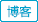#1. 云栖社区>
2. 全部标签>
3. #DataFrame####建模常用的pandas语句

pandas对象是Python常用的数据分析模块，它主要包括series对象，dataframe对象和index对象。每种对象都有自己所特有的方法和属性。今天小编更新下建模中常用的pandas语句

###Python 科学工具使用

Python 科学工具笔记 numpy a = numpy.array([1,2,3,4]);// 创建一个numpy的数组对象 此时a.shape显示的值为(4,); 由此得出结论在一维的数组中, 数组的是列优先的 numpy.

###Python第三方库使用感言

Python第三方库使用的感言加使用笔记 一般来讲第三方库会提供大量的类与对象, 对象方法的返回值和库中函数的返回值一般不会是Python原始自带的对象, 而是由该第三方库提供的对象, 因为Python的开源性, 第三方库的编写风格各异, 不怎么好记忆, 如, 一般常理来说, 函数应该是动词, 而在matplotlib.

###Python 科学工具笔记

Python 科学工具笔记 numpy a = numpy.array([1,2,3,4]);// 创建一个numpy的数组对象 此时a.shape显示的值为(4,); 由此得出结论在一维的数组中, 数组的是列优先的 numpy.

25
GO